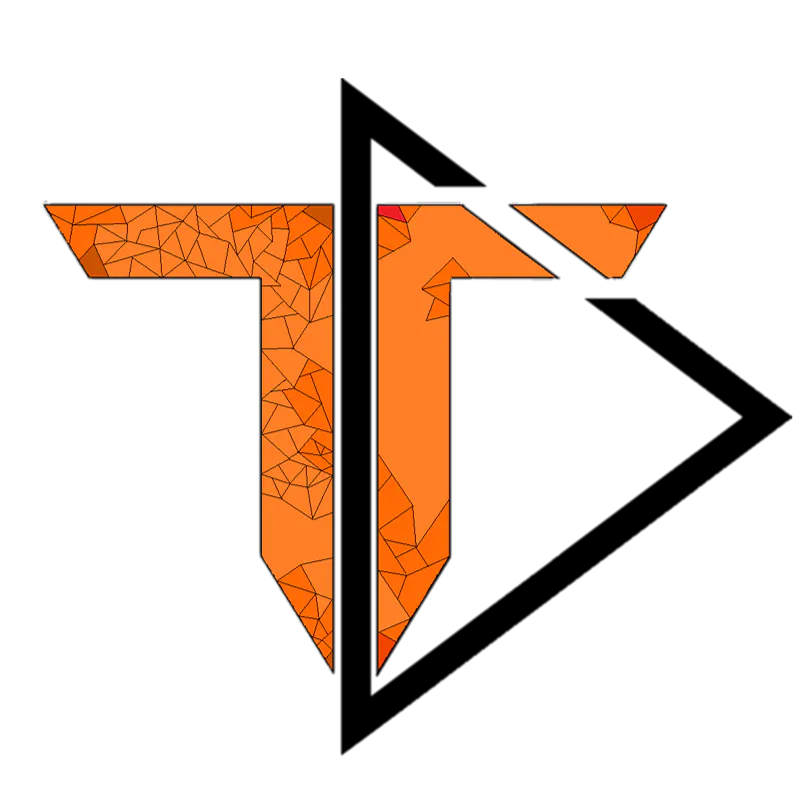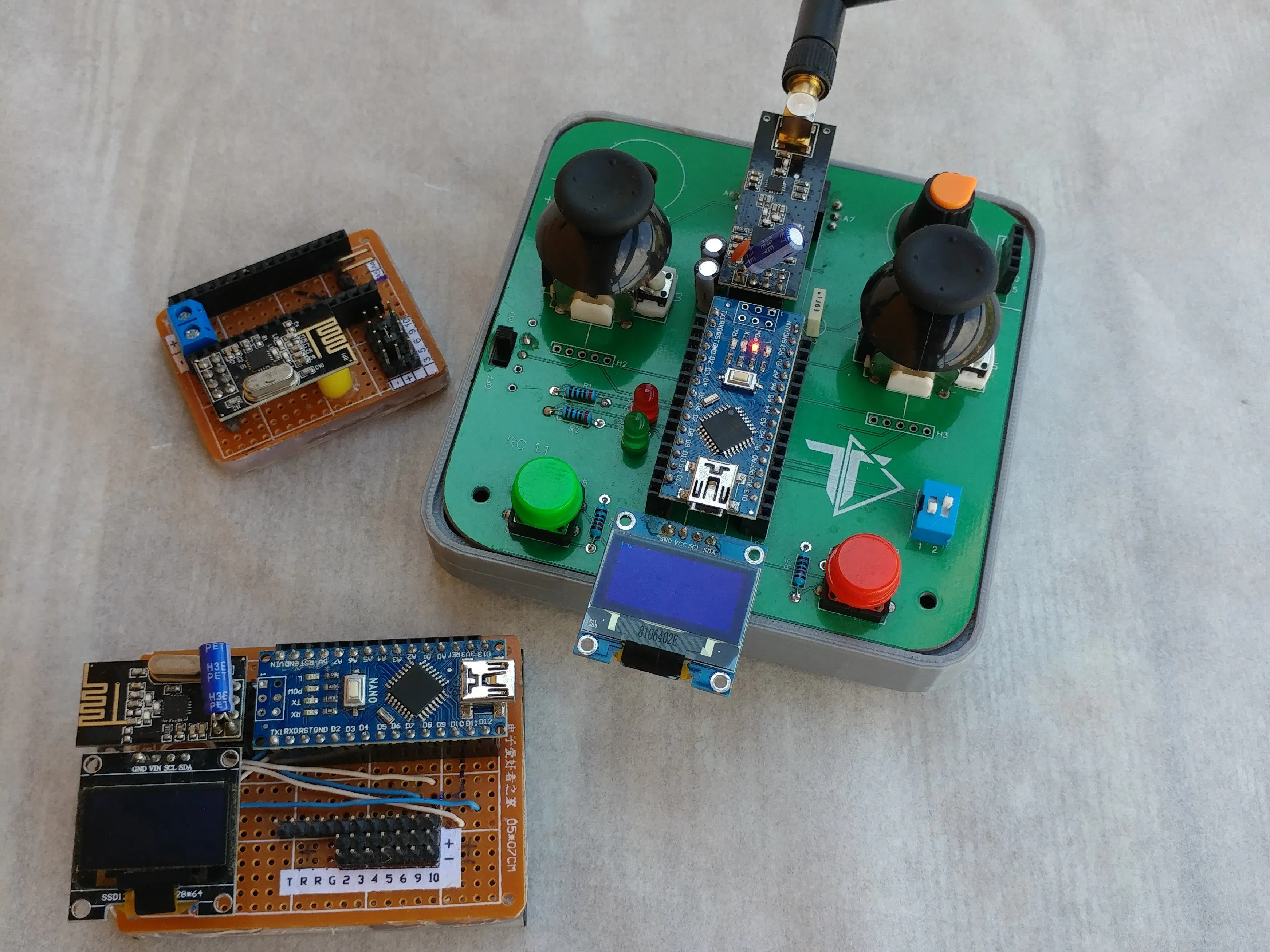## Things used in this project

### Hardware componentsArduino Nano R3
×1

### Software apps and online servicesArduino IDE

## Code

### nrf24l01 remote

Arduino
```//Remote Transmitter
#include <SPI.h>
#include <nRF24L01.h>
#include <RF24.h>

//LED Display
#include <Wire.h>

#define L_AXIS A0 // Left Y Axis Port
#define R_AXIS A2 // Right Y Axis Port
#define Pot A7   // Poteciometer

//LED Display
#define OLED_RESET 5

float value ;
float voltage ;
int percentage;

struct data{
int left;
int right;
int maxs;

};
data send_data;

RF24 radio(7, 8); // CE, CSN
void setup() {

Wire.begin();
display.begin(SSD1306_SWITCHCAPVCC, 0x3C);

}
void loop() {

voltage = ((5*value)/1023)*4.103354632587859 ;
percentage = map((voltage*10), 30, 42, 0, 100);

display.clearDisplay();
display.setTextColor(WHITE);
display.setTextSize(1);

display.setCursor(0,0);
display.print("L= ");
display.print(send_data.left);

display.print("  Power: ");
if( (500 < send_data.left ) && ( send_data.left < 530 ) )  display.println(0);
if( send_data.left <= 500 ){
display.print("-");
display.println(map(send_data.left, 0, 500, 255, 100));
}
if( 530 <= send_data.left )  display.println(map(send_data.left, 530, 1023, 100, 255));

display.print("R= ");
display.print(send_data.right);

display.print("  Power: ");
if( (500 < send_data.right ) && ( send_data.right < 530 ) )  display.print(0);
if( send_data.right <= 500 ){
display.print("-");
display.print(map(send_data.right, 0, 500, 255, 100));
}
if( 530 <= send_data.right )  display.print(map(send_data.right, 530, 1023, 100, 255));

display.print('\n');
display.print('\n');
display.print('\n');
display.print('\n');
display.print("Max Speed: ");
display.print(map(send_data.maxs, 0, 1023, 150, 255));
display.print('\n');
display.print("Voltage: ");
display.print(voltage);
display.print("V   ");
display.print(percentage);
display.print("%");

display.display();

}
```

Arduino
```#include <SPI.h>
#include <nRF24L01.h>
#include <RF24.h>

#include <Wire.h>
#define OLED_RESET 5

//Motor Ports
#define R_FRONT 10
#define R_BACK 9
#define L_FRONT 5
#define L_BACK 6

int maxSpeed ;

const int buzzer = 3; //buzzer to arduino pin 9

RF24 radio(7, 8); // CE, CSN

unsigned long lastRecvTime = 0; //Used for latency check

struct data{
int left;
int right;
int maxs;

};

void reset_the_Data() //Set default values
{

}

{
lastRecvTime = millis();
}
}

void move(char direction[ ], char motor[ ], int value){
if(motor == "right"){
if(direction == "stay"){
analogWrite(R_FRONT,0);
analogWrite(R_BACK,0);
}else if(direction == "backwards"){
analogWrite(R_BACK,map(value, 0, 500, maxSpeed, 100));
}else if(direction == "forwards"){
analogWrite(R_FRONT,map(value, 530, 1023, 100, maxSpeed));
}
}else{
if(direction == "stay"){
analogWrite(L_FRONT,0);
analogWrite(L_BACK,0);
}else if(direction == "backwards"){
analogWrite(L_BACK,map(value, 0, 500, maxSpeed, 100));
}else if(direction == "forwards"){
analogWrite(L_FRONT,map(value, 530, 1023, 100, maxSpeed));
}
maxSpeed = map(receive_data.maxs, 0, 1023, 150, 255);
}

Serial.print(direction);
Serial.print(" ");
Serial.print(motor);
Serial.print(" ");
Serial.println(value);

}

void setup() {

Serial.begin(9600);

Wire.begin();
display.begin(SSD1306_SWITCHCAPVCC, 0x3C);// initialize with the I2C addr 0x3C (for the 128x32)(initializing the display)

}
void loop() {

unsigned long now = millis();
if ( now - lastRecvTime > 1000 ) {  //Check for latency
reset_the_Data();
}

//int value=map(receive_data.right, 0, 1023, 180, 255);
//analogWrite(R_FRONT,value);

display.clearDisplay();
display.setTextColor(WHITE);
display.setTextSize(1);

display.setCursor(0,0);
display.print("LY= ");
display.print("\t");
display.print("  RY= ");

display.display();